## UGC NET CS 2016 Aug- paper-3

 Question 1
A ripple counter is a (n):
 A Synchronous Counter B Asynchronous counter C Parallel counter D None of the above
Digital-Logic-Design       Sequential-Circuits
 Question 2
8085 microprocessor has ____ bit ALU.
 A 32 B 16 C 8 D 4
Computer-Organization       Microprocessor
Question 2 Explanation:
8085 microprocessor has 8 bit ALU. It performs arithmetic and logical operations like Addition, Subtraction, AND, OR, etc. on 8-bit data.
 Question 3
The register that stores the bits required to mask the interrupts is ______.
 A Status register B Interrupt service register C Interrupt mask register D Interrupt request register
Computer-Organization       Interruption
Question 3 Explanation:
Status register(SR):
→ A status register is a collection of status flag bits for a processor.
→ The status register is a hardware register that contains information about the state of the processor. Individual bits are implicitly or explicitly read and/or written by the machine code instructions executing on the processor.
→ The status register lets an instruction take action contingent on the outcome of a previous instruction.
Interrupt service register(ISR):
→ Interrupt service routine(ISR) is a special block of code associated with a specific interrupt condition.
→ Interrupt handlers are initiated by hardware interrupts, software interrupt instructions, or software exceptions, and are used for implementing device drivers or transitions between protected modes of operation, such as system calls.
→ This register enables or masks interrupts from being triggered on the external pins of the Cache Controller.
→ The IMR is used to disable (Mask) or enable (Unmask) individual interrupt request inputs. This is also an 8-bit register.
Interrupt request register(IRR):
→ It stores all the interrupt inputs that are requesting service.
→ It is an 8-bit register, one bit for each interrupt request.
→ It keeps track of which interrupt inputs are asking for service. If an interrupt input is unmasked, and has an interrupt signal on it, then the corresponding bit in the IRR will be set.
→ The content of this register can be read to know the status of pending interrupts.
 Question 4
Which of the following in 8085 microprocessor performs
HL = HL + HL?
Computer-Organization       Microprocessor
 Question 5
In ______ addressing mode, the operands are stored in the memory. The address of the corresponding memory location is given in a register which is specified in the instruction.
 A Register direct B Register indirect C Base indexed D Displacement
Computer-Organization       Interruption
Question 5 Explanation:
Register indirect: It is specified implicitly in the definition of instruction. Register indirect addressing mode, the operands are stored in the memory. The address of the corresponding memory location is given in a register which is specified in the instruction.
Index mode: The address of the operand is obtained by adding to the contents of the general register (called index register) a constant value. The number of the index register and the constant value are included in the instruction code.
 Question 6
The output of the following combinational circuit.A X.Y B X+Y C X⊕Y D (X⊕Y)’
Digital-Logic-Design       Combinational-Circuit
Question 6 Explanation:Question 7
Which of the following statements is/are True regarding some advantages that an object-oriented DBMS (OODBMS) offers over a relational database ?
I. An OODBMS avoids the“impedance mismatch” problem.
II. An OODBMS avoids the “phantom” problem.
III. An OODBMS provides higher performance concurrency control than most relational databases.
IV. An OODBMS provides faster access to individual data objects once they have been read from disk.
 A II and III only B I and IV only C I, II, and III only D I, III and IV only
Database-Management-System       OODBMS
Question 7 Explanation:
TRUE: An OODBMS avoids the“impedance mismatch” problem.
FALSE: An OODBMS avoids the “phantom” problem.
FALSE: An OODBMS provides higher performance concurrency control than most relational databases.
TRUE: An OODBMS provides faster access to individual data objects once they have been read from disk.
 Question 8
The Global conceptual Schema in a distributed database contains information about global relations. The condition that all the data of the global relation must be mapped into the fragments, that is, it must not happen that a data item which belongs to a global relation does not belong to any fragment, is called:
 A Disjointness condition B Completeness condition C Reconstruction condition D Aggregation condition
Database-Management-System       Relational Schema
Question 8 Explanation:
Functional Completeness Condition: It is the condition used to check the equivalence(The condition that all the data of the global relation must be mapped into the fragments) between global relation and its fragments after decomposition. When a relation is decomposed into fragments, both global relation and its fragments should be able to determine each other.
For decomposing a global relation, lossless decomposition is the necessary condition but functionally completeness problem is not a necessary condition.
A relation in BCNF may or may not be functionally complete
 Question 9
Suppose database table T1(P, R) currently has tuples {(10, 5), (15, 8), (25, 6)} and table T2 (A, C) currently has {(10, 6), (25, 3), (10, 5)}. Consider the following three relational algebra queries RA1, RA2 and RA3:The number of tuples in the resulting table of RA1, RA2 and RA3 are given by:
 A 2, 4, 2 respectively B 2, 3, 2 respectively C 3, 3, 1 respectively D 3, 4, 1 respectively
Database-Management-System       Relational-Algebra
Question 9 Explanation:Question 10
Consider the table R with attributes A, B and C. The functional dependencies that hold on R are : A → B, C → AB. Which of the following statements is/are True ?
I. The decomposition of R into R1(C, A) and R2(A, B) is lossless.
II. The decomposition of R into R1(A, B) and R2(B, C) is lossy.
 A Only I B Only II C Both I and II D Neither I nor II
Database-Management-System       Functional-Dependency
Question 10 Explanation:Here ‘B’ is common attribute but ‘B’ is not the primary key in any of the decomposed relations (R1 or R2). Hence this decomposition is not a lossless decomposition i.e., it is a lossy decomposition.
Hence, option (C) is correct.
 Question 11
Consider the following ORACLE relations : One (x, y) = {<2, 5>, <1, 6>, <1, 6>, <1, 6>, <4, 8>, <4, 8>}
Two (x, y) = {<2, 55>, <1, 1>, <4, 4>, <1, 6>, <4, 8>, <4, 8>, <9, 9>, <1, 6>}
Consider the following two SQL queries SQ1 and SQ2 :
SQ1 : SELECT * FROM One)
EXCEPT
(SELECT * FROM Two);
SQ2 : SELECT * FROM One)
EXCEPT ALL
(SELECT * FROM Two);
For each of the SQL queries, what is the cardinality (number of rows) of the result obtained when applied to the instances above ?
 A 2 and 1 respectively B 1 and 2 respectively C 2 and 2 respectively D 1 and 1 respectively
Database-Management-System       SQL
Question 11 Explanation:
EXCEPT operation is like subtraction operation.
EXCEPT : EXCEPT operator do not include duplicates i.e if there are duplicate copies of a tuple in a relation the EXCEPT will consider only one copy of that duplicated tuple.
EXCEPT ALL : EXCEPT operator includes duplicates.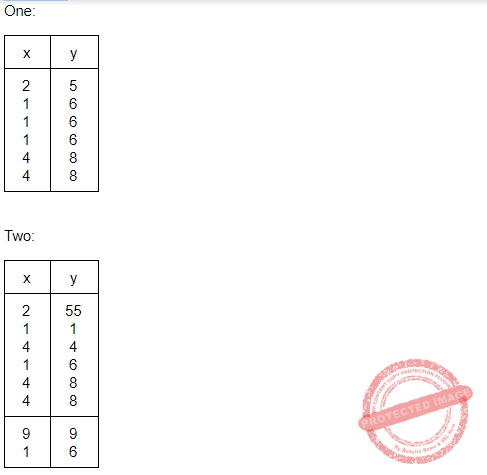Question 12
Which one of the following pairs is correctly matched in the context of database design?A I-D, II-A, III-B, IV-C B I-D, II-C, III-B, IV-A C I-C, II-D, III-A, IV-B D I-C, II-A, III-D, IV-B
Database-Management-System       Databases
Question 12 Explanation:
Specialization: Specialization is a top-down approach, means higher to lower. Result of taking a subset of a higher level entity set to from a lower level entity set.
Generalization: Generalization is a bottom-up approach, means lower to higher. Result of taking the union of two or more disjoint(lower-level) entity sets to produce higher-level entity set.
Aggregation: It is treated as higher level entity sets and can participate in relationships. Mapping cardinalities: Number of entities to which another entity can be associated via a relationship set. An abstraction in which relationship sets(along with their associated entity sets) are treated as higher level entity sets, and can participate in relationships.
Mapping cardinalities: Express the number of entities to which another entity can be associated via a relationship set.
 Question 13
Consider a raster grid having XY-axes in positive X-direction and positive upward Y-direction with Xmax = 10, Xmin = –5, Ymax = 11, and Ymin = 6. What is the address of memory pixel with location (5, 4) in raster grid assuming base address 1 (one) ?
 A 150 B 151 C 160 D 161
Computer-Graphics       Raster-Systems
 Question 14
Consider a N-bit plane frame buffer with W-bit wide lookup table with W > N. How many intensity levels are available at a time ?
 A 2N B 2 W C 2 N+W D 2 N-1
Computer-Graphics       Frame-Buffer
 Question 15
Consider the Bresenham's line generation algorithm for a line with gradient greater than one, current point (xi, yi) and decision parameter, di. The next point to be plotted (xi+1, yi+1) and updated decision parameter, di+1, for di < 0 are given as _______.
 A xi+1 = xi +1 yi+1 = yi di+1 = di+ 2 dy B xi+1 = xi yi+1 = yi +1 di+1 = di+ 2 dx C xi+1 = xi yi+1 = yi +1 di+1 = di+ 2(dx -dy) D xi+1 = xi +1 yi+1 = yi +1 di+1 = di+ 2(dy -dx)
Computer-Graphics       Bresenham’s-Algorithm
Question 15 Explanation: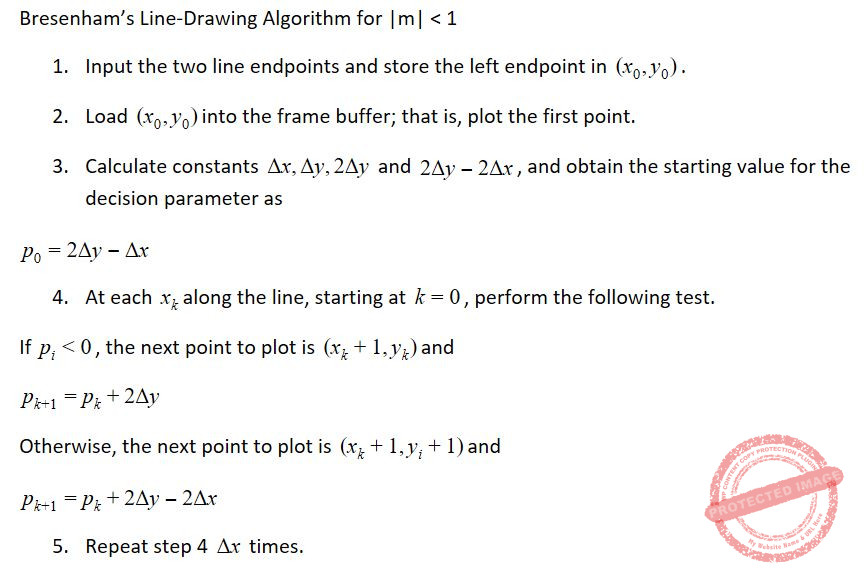Question 16
A point P(2, 5) is rotated about a pivot point (1, 2) by 60°. What is the new transformed point P' ?
 A (1, 4) B (–1, 4) C (1, – 4) D (– 4, 1)
Computer-Graphics       Transformations
Question 16 Explanation: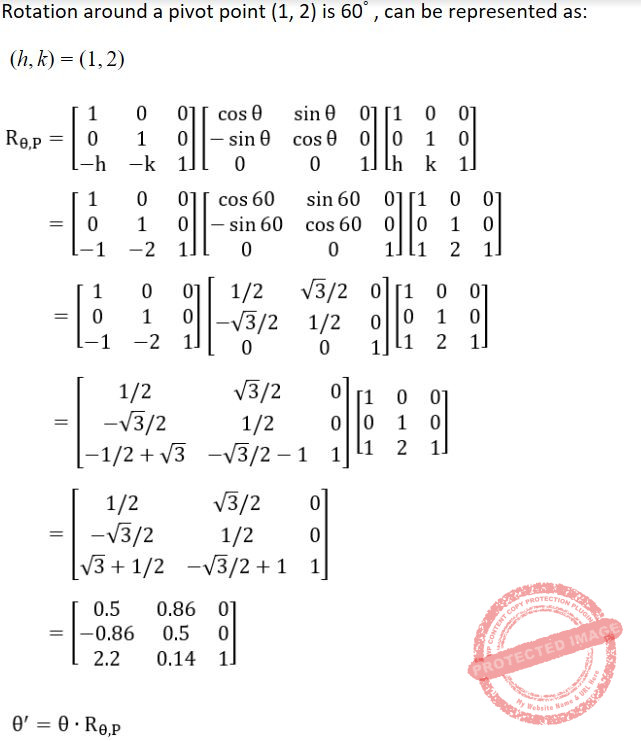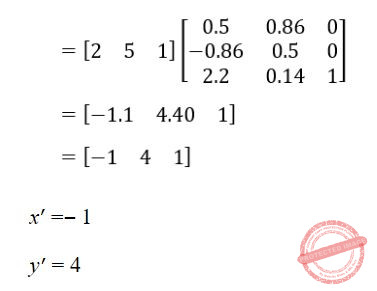Question 17
In perspective projection (from 3D to 2D), objects behind the centre of projection are projected upside down and backward onto the view-plane. This is known as _____.
 A Topological distortion B Vanishing point C View confusion D Perspective foreshortening
Computer-Graphics       3D
 Question 18
The Liang-Barsky line clipping algorithm uses the parametric equation of a line from (x1, y1) to (x2, y2) along with its infinite extension which is given as :
x = x1 + ∆x.u
y = y1 + ∆y.u
Where ∆x = x2– x1, ∆y = y2– y1, and u is the parameter with 0 ≤ u ≤ 1. A line AB with endpoints A(–1, 7) and B(11, 1) is to be clipped against a rectangular window with xmin = 1, xmax = 9, ymin = 2, and ymax = 8.
The lower and upper bound values of the parameter u for the clipped line using Liang-Barsky algorithm is given as :
 A (0, 2/3) B (1/6 , 5/6) C (0, 1/3) D (0, 1)
Computer-Graphics       Liang-Barsky-line-clipping-algorithm
 Question 19
Match the following with reference to Functional programming history:A a-iv, b-i, c-iii, d-ii B a-i, b-iv, c-ii, d-iii C a-iii, b-ii, c-iv, d-i D a-ii, b-i, c-iv, d-iii
Question 19 Explanation:
Lambda calculus→ Church, 1932
Lambda calculus (also written as λ-calculus) is a formal system in mathematical logic for expressing computation based on function abstraction and application using variable binding and substitution. It is a universal model of computation that can be used to simulate any Turing machine. It was first introduced by mathematician Alonzo Church in the 1930s as part of his research of the foundations of mathematics.
Lambda calculus as programming language→ Mccarthy, 1960
The ﬁrst functional programming language and the second oldest programming language still in use (after FORTRAN), LISP began life in 1958 as a project led by John McCarthy at MIT. The aim was to create a system for programming computations over symbolic data, starting with an algorithm McCarthy had drafted for symbolic diﬀerentiation.
Lazy evaluation→ Wordsworth, 1970
Lazy evaluation was introduced for lambda calculus by Christopher Wadsworth. lazy evaluation (or) call-by-need is an evaluation strategy which delays the evaluation of an expression until its value is needed (non-strict evaluation) and which also avoids repeated evaluations (sharing).
Type classes, as used in the functional programming language Haskell.
 Question 20
Aliasing in the context of programming languages refers to:
 A Multiple variables having the same location B Multiple variables having the same identifier C Multiple variables having the same value D Multiple use of same variable
Question 20 Explanation:
In computer programming, aliasing refers to the situation where the same memory location can be accessed using different names.
 Question 21
Assume that the program ‘P’ is implementing parameter passing with ‘call by reference’. What will be printed by following print statements in P?
Program P( )
{
x = 10;
y = 3;
funb (y, x, x)
print x;
print y;
}
funb (x, y, z)
{
y = y + 4;
z = x + y + z;
}
 A 10, 7 B 31, 3 C 10, 3 D 31, 7
Programming       Pointers
Question 21 Explanation:
Here, variable x of func1 points to address of variable y.
And variable y and z of func1 points to address of variable x.
Therefore, y = y+4 ⇒ y = 10+4 = 14
and z = x+y+z ⇒ z = 14+14+3 = 31
z will be stored back in k.
Hence, x=31 and y will remain as it is (y=3).
 Question 22
The regular grammar for the language L = {anbm | n + m is even} is given by
 A S → S1 | S2 S1 → a S1| A1 A1 → b A1| λ S2 → aaS2| A2 A2 → b A2| λ B S → S1 | S2 S1 → a S1| aA1 S2 → aaS2 | A2 A1 → b A1| λ A2 → b A2| λ C S → S1 | S2 S1 → aaa S1| aA1 S2 → aaS2| A2 A1 → b A1| λ A2 → b A2| λ D S → S1 | S2 S1 → aa S1 | A1 S2 → aaS2 | aA2 A1 → bbA1 | λ A2 → bbA | b
Theory-of-Computation       Regular-Language
Question 22 Explanation:
NOTE: Here "λ" means NULL.
Option(A) is not correct because it can generate strings like { aab, aaaab, aaaaaab,............}
S → S1
S1 → a S1
S1 → aa S1
S1 → aaA1
S1 → aab A1
S1 → aabλ
Option(B) is not correct because it can generate strings like { aab, aaaab, aaaaaab,............}
S → S1
S1 → a S1
S1 → aaA1
S1 → aabA1
S1 → aabλ
Option(C) is not correct because it can generate strings like { aab, aaaab, aaaaaab,............}
S → S2
S2 → aaS2
S2 → aaA2
S2 → aabA2| λ
S2 → aabλ
Option(D) is correct because it generates strings like {ab, aabb, aaab,..........}
 Question 23
Let Σ = {a, b} and language L = {aa, bb}. Then, the complement of L is
 A {λ, a, b, ab, ba} ∪ {w∈{a, b}* | |w| > 3} B {a, b, ab, ba} ∪ {w∈{a, b}* | |w| > 3} C {w ∈ { a, b}* | |w| > 3} ∪ {a, b, ab, ba} D {λ, a, b, ab, ba} ∪ {w ∈ {a, b}* | |w| ≥ 3}
Theory-of-Computation       Languages-and-Grammars
Question 23 Explanation:
Complement of a language L means the complement form of given language L will contain all String except the strings that L contains.
Here L= {aa,bb}
It means complement of L can contain { λ, a, b, ab, ba, aaa, aab, aba, bba,..................}
Hence Option (D) is correct.
 Question 24
Consider the following identities for regular expressions:
(a) (r + s)* = (s + r)*
(b) (r*)* = r*
(c) (r* s*)* = (r + s)*
Which of the above identities are true?
 A (a) and (b) only B (b) and (c) only C (c) and (a) only D (a), (b) and (c)
Theory-of-Computation       Regular-Expression
Question 24 Explanation:
The given identities are the closure properties of regular expressions. Following are few identities for regular expressions:
∅* = ε
ε* = ε
RR* = R*R
R*R* = R*
(R*)* = R*
RR* = R*R
(PQ)*P =P(QP)*
(r+s)* = (r*s*)* = (r*+s*)* = r*(sr*)*
Hence option(D) is correct.
 Question 25
Suppose transmission rate of a channel is 32 kbps. If there are ‘8’ routes from source to destination and each packet p contains 8000 bits. Total end to end delay in sending packet P is _____
 A 2 sec B 3 sec C 4 sec D 1 sec
Computer-Networks       Routing
Question 25 Explanation:
Given data,
-- Transmission rate of a channel= 32Kbps
-- Total routes= 8
-- Packet ‘p’= 8000 bits
-- Total end to end delay=?
Step-1: Total end to end delay= (Total Routes * Packets ‘p’) / Transmission rate of a channel
= 8 * 8000 bits / 32 (Note: 1 kbps= 1024)
= 8 * 8000 bits / 32000 bps
= 2 sec
 Question 26
Consider the following statements :
A. High speed Ethernet works on optic fiber.
B. A point to point protocol over Ethernet is a network protocol for encapsulating PPP frames inside Ethernet frames.
C. High speed Ethernet does not work on optic fiber.
D. A point to point protocol over Ethernet is a network protocol for encapsulating Ethernet frames inside PPP frames.
Which of the following is correct ?
 A A and B are true; C and D are false. B A and B are false; C and D are true. C A, B, C and D are true. D A, B, C and D are false.
Computer-Networks       Ethernet
Question 26 Explanation:
TRUE: High speed Ethernet works on optic fiber.
TRUE: A point to point protocol over Ethernet is a network protocol for encapsulating PPP frames inside Ethernet frames.
FALSE: High speed Ethernet does not work on optic fiber.
FALSE: A point to point protocol over Ethernet is a network protocol for encapsulating Ethernet frames inside PPP frames.
 Question 27
In CRC checksum method, assume that given frame for transmission is 1101011011 and the generator polynomial is G(x) = x4 + x + 1. After implementing CRC encoder, the encoded word sent from sender side is _____.
 A 11010110111110 B 11101101011011 C 11101101011011 D 110101111001111
Computer-Networks       Error-Control-Methods
Question 27 Explanation:Question 28
A slotted ALOHA network transmits 200 bits frames using a shared channel with 200 kbps bandwidth. If the system (all stations put together) produces 1000 frames per second, then the throughput of the system is _______.
 A 0.268 B 0.468 C 0.368 D 0.568
Computer-Networks       Access-Control-Methods
Question 28 Explanation:
Step-1: Given data,
Slotted ALOHA transmits=200 bit frames
Bandwidth=200 Kbps

System produces=1000 frames per second
Throughput=?
Step-2: Slotted ALOHA formula S= G*e-G
Frame transmission time=200/200 kbps=1ms
Here, G=1 and S=G*e-G
=0.368 (or) 36.8%
System produces throughput =1000*0.368
=368 frames
 Question 29
An analog signal has a bit rate of 8000 bps and a baud rate of 1000.
Then analog signal has _____ signal elements and carry _____ data elements in each signal.
 A 256, 8 bits B 128, 4 bits C 256, 4 bits D 128, 8 bits
Question 29 Explanation:
Given data,
-- Bit rate(B)=8000 bps
-- Baud rate(Ba)=1000 bps
-- Signal elements(S)=?
-- Data elements(D)=?
Step-1: Total number of signal elements(S)⇒ Ba=B*(1/S) ⇒ S=(B/Ba)
= 8000/1000
= 8 bits/baud
Step-2: Total number of data elements(D) ⇒ S=log2D ⇒ D=2S
=28
= 256 bits
 Question 30
The plain text message BAHI encrypted with RSA algorithm using e = 3, d = 7 and n = 33 and the characters of the message are encoded using the values 00 to 25 for letters A to Z. Suppose character by character encryption was implemented. Then, the Cipher Text message is _____.
 A ABHI B HAQC C IHBA D BHQC E None of the above
Computer-Networks       RSA-algorithm
Question 30 Explanation:
Given data,
-- Message are encoded using values= 00 to 25(It means A=00,B=01,...Z=25)
-- e=3
-- d=7
-- n=33
-- Plain text message(P)=BAHI
-- Cipher text message=Pd mod n=?Question 31
Consider the problem of a chain < A1, A2, A3, A4 > of four matrices. Suppose that the dimensions of the matrices A1, A2, A3 and A4 are 30 × 35, 35 × 15, 15 × 5 and 5 × 10 respectively. The minimum number of scalar multiplications needed to compute the product A1A2A3A4 is ____.
 A 14875 B 21000 C 9375 D 11875
Algorithms       Dynamic-Programming
Question 31 Explanation:Question 32
Consider a hash table of size m = 10000, and the hash function h(K) = floor (m(KA mod1)) for A = ( √(5) – 1)/2. The key 123456 is mapped to location ______.
 A 46 B 41 C 43 D 48
Data-Structures       Hashing
Question 32 Explanation:
Given data,
-- Hash table size(m)=10000
-- Hash function(h(K))= floor (m(KA mod1))
-- A=(√(5)-1)/2
-- key(K)=123456
-- location=?
Step-1: Hash function(h(K))= floor (m(KA mod1))
h(123456) = floor(10000 * (123456 * (√5 − 1) / 2) mod 1)
= floor(10000 * (76300.004115 mod 1)
= floor(10000 * (.004115))
= floor(41.15)
= 41
 Question 33
Consider a weighted complete graph G on the vertex set {ν1, ν2, .... νn} such that the weight of the edge (νi, νj) is 4 | i – j|. The weight of minimum cost spanning tree of G is :
 A 4n2 B n C 4n-4 D 2n-2
Algorithms       Minimum-Spanning-Tree
Question 33 Explanation:
1) Including the initial call which means write the length of the spanning tree, a simple one it is. (Based on a particular graph)
2)Weight of the minimum spanning tree
= 4|2 - 1| + 4|3 - 2| + 4|4 - 3| + 4|5 - 4| .... + 4| n - (n-1) |
= 4n - 4
 Question 34
A priority queue is implemented as a max-heap. Initially, it has ve elements. The level-order traversal of the heap is as follows:  20, 18, 15, 13, 12  Two new elements ‘10’ and ‘17’are inserted in the heap in that order. The level-order traversal of the heap after the insertion of the element is:
 A 20, 18, 17, 15, 13, 12, 10 B 20, 18, 17, 12, 13, 10, 15 C 20, 18, 17, 10, 12, 13, 15 D 20, 18, 17, 13, 12, 10, 15
Data-Structures       Heap-Tree
Question 34 Explanation:Question 35
If there are n integers to sort, each integer has d digits, and each digit is in the set {1, 2, ...,k}, radix sort can sort the numbers in:
 A O (k (n + d)) B O(d (n + k)) C O((n + k) lg d) D O((n + d) lg k)
Algorithms       Sorting
Question 35 Explanation: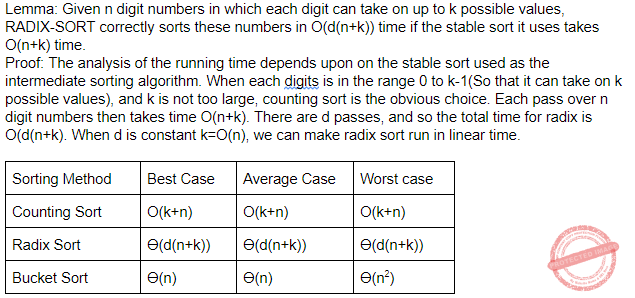Question 36
Match the following:A a-i, b-iii, c-iv, d-ii B a-i, b-iii, c-ii, d-iv C a-iii, b-i, c-iv, d-ii D a-iii, b-i, c-ii, d-iv
Algorithms       Greedy-approach
Question 36 Explanation:
Prim’s algorithm→ O(ElogV)
Bellman-Ford algorithm→ O(V2E)
Floyd-Warshall algorithm→ O(V3)
Johnson’s algorithm→ O(VE lg V)
 Question 37
Constructors have _____ return type.
 A void B char C int D no
Programming-in-c++       Constructor-Destructor
Question 37 Explanation:
Syntax For Constructors:
Access_Modifier No Return_Type Class(...)
Example:
public static main(String [ ]args)
Constructor rules:
1. Constructors are always used with a new.
2. Have same name as that of Class
3. Does not have a return type
4. Can be Overloaded but not Overridden.
 Question 38
Method overriding can be prevented by using final as a modifier at ______.
 A The start of the class. B The start of method declaration. C The start of derived class. D The start of the method declaration in the derived class.
OOPS       Properties
Question 38 Explanation:
Method overriding can be prevented by using final as a modifier at the start of method declaration.
 Question 39
Which of the following is a correct statement?
 A Composition is a strong type of association between two classes with full ownership. B Composition is a strong type of association between two classes with partial ownership. C Composition is a weak type of association between two classes with partial ownership. D Composition is a weak type of association between two classes with strong ownership.
OOPS       Class-and-object
Question 39 Explanation:Question 40
Which of the following is not a correct statement?
 A Every class containing abstract method must be declared abstract. B Abstract class can directly be initiated with ‘new’ operator. C Abstract class can be initiated. D Abstract class does not contain any de nition of implementation.
OOPS       Class-and-object
Question 40 Explanation:
A class which is declared as abstract is known as an abstract class. It can have abstract and non-abstract methods. It needs to be extended and its method implemented. It cannot be instantiated.
Rules:
1. An abstract class must be declared with an abstract keyword.
2. It can have abstract and non-abstract methods.
3. It cannot be instantiated.
4. It can have constructors and static methods also.
5. It can have final methods which will force the subclass not to change the body of the method.
 Question 41
Which of the following tag in HTML is used to surround information, such as signature of the person who created the page ?
 A < body > < / body > B < address > < / address > C < strong > < / strong > D < em > < / em >
Web-Technologies       HTML
Question 41 Explanation:
This tag is for address information, signatures, etc, normally at the top or bottom of a document. typically, it is italic and/or right justified or indented.
The format is:
 Question 42
Java uses threads to enable the entire environment to be ______.
 A Symmetric B Asymmetric C Synchronous D Asynchronous
OOPS       JAVA
Question 42 Explanation:
→ Java uses threads to enable the entire environment to be asynchronous.
→ Synchronous (or) Synchronized means "connected", or "dependent" in some way. In other words, two synchronous tasks must be aware of one another, and one task must execute in some way that is dependent on the other, such as wait to start until the other task has completed.
→ Asynchronous means they are totally independent and neither one must consider the other in any way, either in initiation or in execution.
 Question 43
An Operating System (OS) crashes on the average once in 30 days, that is, the Mean Time Between Failures (MTBF) = 30 days. When this happens, it takes 10 minutes to recover the OS, that is, the Mean Time To Repair (MTTR) = 10 minutes. The availability of the OS with these reliability figures is approximately :
 A 96.97% B 97.97% C 99.009% D 99.97%
Software-Engineering       Software-Reliabiliy
Question 43 Explanation:
Given data,
-- Mean Time Between Failures (MTBF) = 30 days
-- Mean Time to Repairs (MTTR) = 10 minutes
-- Availability=?
Step-1: Given repair time in minutes, so we have to convert failure time in minutes.
30 days= 30 ∗ 24 ∗ 60 minutes
= 43,200 minutes
Step-2: Given options in percentages. We have to find availability in percentages.
Availability=(100-((10 / 43,200)∗100))%
= 100-0.023%
= 99.97%
 Question 44
Match each software lifecycle model in List – I to its description in List – II:A I-e, II-b, III-a, IV-c, V-d B I-e, II-c, III-a, IV-b, V-d C I-d, II-a, III-b, IV-c, V-e D I-c, II-e, III-a, IV-b, V-d
Software-Engineering       Types-of-Models
Question 44 Explanation:
Code and Fix → Write some code, debug it, repeat(i.e. ad-hoc)
Evolutionary prototype → Build an initial small requirement specifications, code it, then "evolve" the specifications and code as needed.
Spiral → Assess risks at each step; do most critical action first.
Staged Delivery→ Build initial requirement specification for several releases, then design-and-code in sequence
Waterfall → Standard phases(requirements,design,code,test) in order.
 Question 45
Match each software term in List – I to its description in List – II: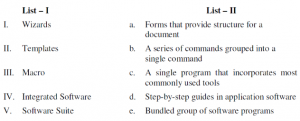A I-d, II-a, III-b, IV-c, V-e B I-b, II-a, III-d, IV-c, V-e C I-d, II-e, III-b, IV-a, V-c D I-e, II-c, III-b, IV-a, V-d
Software-Engineering       Types-of-Models
Question 45 Explanation:
Wizards→ Step-by-step guides in application software
Templates→ Forms that provide structure for a document
Macro→ A series of commands grouped into a single command
Integrated Software→ A single program that incorporates most commonly used tools
Software Suite→ Bundled group of software programs
 Question 46
The ISO quality assurance standard that applies to software Engineering is
 A ISO 9000 : 2004 B ISO 9001 : 2000 C ISO 9002 : 2001 D ISO 9003 : 2004
Software-Engineering       Software-quality
Question 46 Explanation:
→ Software quality assurance (SQA) consists of a means of monitoring the software engineering processes and methods used to ensure quality.
→ The ISO quality assurance standard that applies to software Engineering is ISO 9001 : 2000.
 Question 47
Which of the following are external qualities of a software product?
 A Maintainability, reusability, portability, efficiency, correctness. B Correctness, reliability, robustness, efficiency, usability. C Portability, interoperability, maintainability, reusability. D Robustness, efficiency, reliability, maintainability, reusability.
Software-Engineering       Software-quality
Question 47 Explanation:
Steve McConnell’s Code describes the characteristics of internal and external qualities
1. External Quality Characteristics: Correctness, Usability, Efficiency, Reliability, Integrity, Adaptability, Accuracy, and Robustness.
2. Internal Quality Characteristics: Maintainability, Flexibility, Portability, Re-usability, Readability, Testability, and Understandability.
 Question 48
Which of the following is/are CORRECT statement(s) about version and release ?
I. A version is an instance of a system, which is functionally identical but non-functionally distinct from other instances of a system.
II. A version is an instance of a system, which is functionally distinct in some way from other system instances.
III. A release is an instance of a system, which is distributed to users outside of the development team.
IV. A release is an instance of a system, which is functionally identical but non-functionally distinct from other instances of a system.
 A I and III B II and IV C I and IV D II and III
Question 48 Explanation:
TRUE: A version is an instance of a system, which is functionally distinct in some way from other system instances.
TRUE: A release is an instance of a system, which is distributed to users outside of the development team.
Build, version and Release terminology:
Build − executable or a library created by compiling source code.
Version − a software build. New version is a different build.
Release − (public release) a version intended for use by general population.
 Question 49
The Unix Operating System Kernel maintains two key data structures related to processes, the process table and the user structure. Now, consider the following two statements :
I. The process table is resident all the time and contain information needed for all processes, even those that are not currently in memory.
II. The user structure is swapped or paged out when its associated process is not in memory, in order not to waste memory on information that is not needed.
Which of the following options is correct with reference to above statements ?
 A Only (I) is correct. B Only (II) is correct. C Both (I) and (II) are correct. D Both (I) and (II) are wrong.
Operating-Systems       UNIX-Operating-System
Question 49 Explanation:
TRUE: The process table is resident all the time and contain information needed for all processes, even those that are not currently in memory.
TRUE: The user structure is swapped or paged out when its associated process is not in memory, in order not to waste memory on information that is not needed.
 Question 50
Consider a system which have ‘n’ number of processes and ‘m’ number of resource types.The time complexity of the safety algorithm, which checks whether a system is in safe state or not, is of the order of:
 A O(mn) B O(m2 n2 ) C O(m2 n) D O(mn2 )
Question 50 Explanation:
Safety algorithm:
1) Let Work and Finish be vectors of length ‘m’ and ‘n’ respectively.
Initialize: Work = Available
Finish[i] = false; for i=1, 2, 3, 4….n
2) Find an i such that both
a) Finish[i] = false
b) Needi <= Work
if no such i exists goto step (4)
3) Work = Work + Allocation[i]
Finish[i] = true
goto step (2)
4) if Finish [i] = true for all i

→ The time complexity of the safety algorithm, which checks whether a system is in safe state or not, is of the order of O(mn2).
Where, ‘n’ number of processes and ‘m’ number of resource types.
 Question 51
An operating system supports a paged virtual memory, using a central processor with a cycle time of one microsecond. It costs an additional one microsecond to access a page other than the current one. Pages have 1000 words, and the paging device is a drum that rotates at 3000 revolutions per minute and transfers one million words per second.Further, one percent of all instructions executed accessed a page other than the current page. The instruction that accessed another page, 80% accessed a page already in memory and when a new page was required, the replaced page was modi ed 50% of the time. What is the effective access time on this system, assuming that the system is running only one process and the processor is idle during drum transfers?
 A 30 microseconds B 34 microseconds C 60 microseconds D 68 microseconds
Operating-Systems       Virtual Memory
 Question 52
Consider the following page reference string :  1, 2, 3, 4, 2, 1, 5, 6, 2, 1, 2, 3, 7, 6, 3, 2, 1, 2, 3,6  Which of the following options, gives the correct number of page faults related to LRU,FIFO, and optimal page replacement algorithms respectively, assuming 05 page frames and all frames are initially empty ?
 A 10, 14, 8 B 8, 10, 7 C 7, 10, 8 D 7, 10, 7
Operating-Systems       Page-Replacement-algorithm
Question 52 Explanation: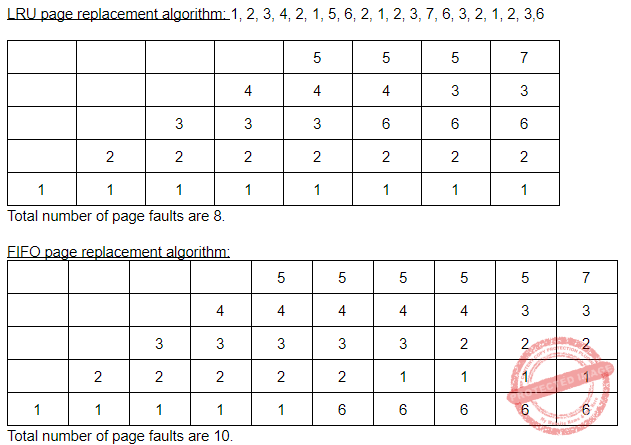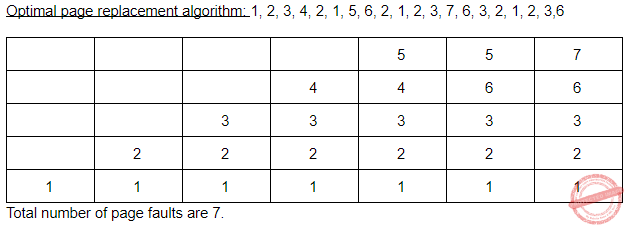Question 53
Consider a file currently consisting of 50 blocks. Assume that the file control block and the index block is already in memory. If a block is added at the end (and the block information to be added is stored in memory), then how many disk I/O operations are required for indexed (single-level) allocation strategy?
 A 1 B 101 C 27 D 0
Operating-Systems       File system-I/O-protection
Question 53 Explanation:
Given data,
-- Up time=75%
-- Down time=25%
-- How many times does this file server have to be replicated to give an Availability of at least 99% is=?
Option-A: Here 2 replications, it means down time is 25% equivalent 0.25.
According to 2 replications we have to multiply with 0.25*0.25=0.0625
Availability= 100-6.25%
= 93%
Option-B: Here 4 replications, it means down time is 25% equivalent 0.25.
According to 4 replications we have to multiply with 0.25*0.25*0.25*0.25
= 0.00390625
Availability= 100-0.0390625%
= 99.9609375%
Option-C: Here 8 replications, it means down time is 25% equivalent 0.25.
According to 8 replications we have to multiply with
= 0.25*0.25*0.25*0.25*0.25*0.25*0.25*0.25
= 0.0000152587890625
Availability= 100-0.00152587890625%
= 99.99847412109375%
Option-D: Here 16 replications, it means down time is 25% equivalent 0.25.
According to 16 replications we have to multiply with
= 0.25*0.25*0.25*0.25*0.25*0.25*0.25*0.25*0.25*0.25*0.25*0.25*0.25*0.25*0.25*0.25
= 0.00000000023283064365386962890625
Availability= 100-0.000000023283064365386962890625%
= 99.999999976716935634613037109375%
Hence, option-B is most suitable answer.
 Question 54
Given the following two languages :
L1 = {uwwRν | u, v, w ∈ {a, b}+}
L2 = {uwwRν | u, ν, w ∈ {a, b}+, |u| > |ν|}
Which of the following is correct ?
 A L1 is regular language and L2 is not regular language. B L1 is not regular language and L2 is regular language. C Both L1 and L2 are regular languages. D Both L1 and L2 are not regular languages.
Theory-of-Computation       Regular-Language
Question 54 Explanation:Question 55
An experimental file server is up 75% of the time and down for 25% of the time due to bugs. How many times does this file server have to be replicated to give an availability of at least 99% ?
 A 2 B 4 C 8 D 16
Question 55 Explanation:
Given data,
-- Up time=75%
-- Down time=25%
-- How many times does this file server have to be replicated to give an Availability of at least 99% is=?
Option-A: Here 2 replications, it means down time is 25% equivalent 0.25.
According to 2 replications we have to multiply with 0.25*0.25=0.0625
Availability= 100-6.25%
= 93%
Option-B: Here 4 replications, it means down time is 25% equivalent 0.25.
According to 4 replications we have to multiply with 0.25*0.25*0.25*0.25
= 0.00390625
Availability= 100-0.0390625%
= 99.9609375%
Option-C: Here 8 replications, it means down time is 25% equivalent 0.25.
According to 8 replications we have to multiply with
= 0.25*0.25*0.25*0.25*0.25*0.25*0.25*0.25
= 0.0000152587890625
Availability= 100-0.00152587890625%
= 99.99847412109375%
Option-D: Here 16 replications, it means down time is 25% equivalent 0.25.
According to 16 replications we have to multiply with
= 0.25*0.25*0.25*0.25*0.25*0.25*0.25*0.25*0.25*0.25*0.25*0.25*0.25*0.25*0.25*0.25
= 0.00000000023283064365386962890625
Availability= 100-0.000000023283064365386962890625%
= 99.999999976716935634613037109375%
Hence, option-B is most suitable answer.
 Question 56
Given a Turing Machine  M = ({q 0 , q 1 }, {0, 1}, {0, 1, B}, δ, B, {q1 })  Where δ is a transition function de ned as  δ(q 0 , 0) = (q 0 , 0, R)  δ(q 0 , B) = (q 1 , B, R)  The language L(M) accepted by Turing machine is given as :
 A 0* 1* B 00* C 10* D 1*0*
Theory-of-Computation       Turing-machines
Question 56 Explanation:Question 57
Let G = (V, T, S, P) be a context-free grammar such that every one of its productions is of the form A → ν, with |ν| = k > 1. The derivation tree for any string W ∈ L (G) has a height such that
 A h<((|W| - 1)/k-1) B logk|W| < h C logk|W| < h < ((|W| - 1)/k-1) D logk|W| <= h <= ((|W| - 1)/k-1)
Theory-of-Computation       Context-Free-Grammar
Question 57 Explanation:
Min height of any tree is logk|W|
For max height (since k>1, consider k=2) , hence it is CNF form and in CNF height is log2|W|+1)
So, in general it should be logk((|w|-1) / (k-1))
 Question 58
Which of the following is not used in standard JPEG image compression?
 A Huffman coding B Run length encoding C Zig-zag scan D K-L Transform
Image-Processing       Image-Compression
Question 58 Explanation:
→ Huffman coding, Run length encoding and ZIg-zag scan is used to compress the image.
K-L Transform is a representation of a stochastic process as an infinite linear combination of orthogonal functions, analogous to a Fourier series representation of a function on a bounded interval. The transformation is also known as Hotelling transform and eigenvector transform, and is closely related to principal component analysis (PCA) technique widely used in image processing and in data analysis in many fields.
 Question 59
Which of the following is a source coding technique?
 A Huffman coding B Arithmetic coding C Run-length coding D DPCM
Question 59 Explanation:
→ Huffman coding, Arithmetic coding and ZIg-zag scan is used to compress the image/text.
→ Differential pulse-code modulation (DPCM) is a signal encoder that uses the baseline of pulse-code modulation (PCM) but adds some functionalities based on the prediction of the samples of the signal. The input can be an analog signal or a digital signal.
 Question 60
If the histogram of an image is clustered towards origin on X-axis of a histogram plot then it indicates that the image is ______.
 A Dark B Good contrast C Bright D Very low contrast
Image-Processing       Histogram
Question 60 Explanation:
→ If the histogram of an image is clustered towards origin on X-axis of a histogram plot then it indicates that the image is dark.
→ An image histogram is a type of histogram that acts as a graphical representation of the tonal distribution in a digital image. It plots the number of pixels for each tonal value. By looking at the histogram for a specific image a viewer will be able to judge the entire tonal distribution at a glance.
→ The histogram for a very dark image will have the majority of its data points on the left side and center of the graph. Conversely, the histogram for a very bright image with few dark areas and/or shadows will have most of its data points on the right side and center of the graph.
 Question 61
Consider the following linear programming problem :
Max. z = 0.50 x2 – 0.10x1
Subject to the constraints
2x1 + 5x2 < 80
x1 + x2 < 20
and x1, x2 > 0
The total maximum profit (z) for the above problem is :
 A 6 B 8 C 101 D 12
LPP       Maximum-Profit
 Question 62
Consider the following statements :
(a) If primal (dual) problem has a finite optimal solution, then its dual (primal) problem has a finite optimal solution.
(b) If primal (dual) problem has an unbounded optimal solution, then its dual (primal) has no feasible solution at all.
(c) Both primal and dual problems may be infeasible.
Which of the following is correct ?
 A (a) and (b) only B (a) and (c) only C (b) and (c) only D (a), (b) and (c)
LPP       Duality
 Question 63
Consider the following statements :
(a) Assignment problem can be used to minimize the cost.
(b) Assignment problem is a special case of transportation problem.
(c) Assignment problem requires that only one activity be assigned to each resource.
Which of the following options is correct ?
 A (a) and (b) only B (a) and (c) only C (b) and (c) only D (a), (b) and (c)
LPP       Assignment-Problem
 Question 64
What are the following sequence of steps taken in designing a fuzzy logic machine?
 A Fuzzi cation → Rule evaluation → Defuzzi cation B Fuzzi cation → Defuzzi cation → Rule evaluation C Rule evaluation → Fuzzi cation → Defuzzi cation D Rule evaluation → Defuzzi cation → Fuzzi cation
Artificial-intelligence       Fuzzy-sets
Question 64 Explanation:Question 65
Which of the following 2 input Boolean logic functions is linearly inseparable ?
(a) AND
(b) OR
(c) NOR
(d) XOR
(e) NOT XOR
 A (a) and (b) B (b) and (c) C (c), (d) and (e) D (d) and (e)
Digital-Logic-Design       Logic-Gates
 Question 66
Let R and S be two fuzzy relations defined asThen, the resulting relation, T, which relates elements of universe of X to elements of universe of Z using max-product composition is given by
 ABC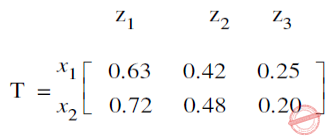DArtificial-intelligence       Fuzzy-sets
Question 66 Explanation:Question 67
Consider the following operations to be performed in Unix :
“The pipe sorts all files in the current directory modified in the month of “June” by order of size and prints them to the terminal screen. The sort option skips ten fields then sorts the lines in numeric order.”
Which of the following Unix command will perform above set of operations ?
 A ls – l | grep “June” | sort +10n B ls – l | grep “June” | sort +10r C ls – l | grep – v “June” | sort +10n D ls – l | grep – n “June” | sort +10x
Operating-Systems       UNIX-Operating-System
Question 67 Explanation:
ls → It will display all files in a current directory.
ls -l → It will display full details(like permissions,links,size,type of file,file name,date of modification)
grep(global search for the regular expression) → The grep command is a filter that is used to search for lines matching a specified pattern and print the matching lines to standard output. sort → To sort either ascending order/descending order. By default it sorts ascending order. sort -n → To sort a file numerically used –n option. -n option is also predefined in unix as the above options are. This option is used to sort the file with numeric data present inside.
 Question 68
Which of the following statements is incorrect for a Windows Multiple Document Interface (MDI) ?
 A Each document in an MDI application is displayed in a separate child window within the client area of the application’s main window. B An MDI application has three kinds of windows namely a frame window, an MDI client window and number of child windows. C An MDI application can support more than one kind of document. D An MDI application displays output in the client area of the frame window.
Operating-Systems       Windows-Operating-System
Question 68 Explanation:
A multiple document interface (MDI) is a graphical user interface in which multiple windows reside under a single parent window. Such systems often allow child windows to embed other windows inside them as well, creating complex nested hierarchies. This contrasts with single document interfaces (SDI) where all windows are independent of each other.
Properties:
1. Each document in an MDI application is displayed in a separate child window within the client area of the application’s main window.
2. An MDI application has three kinds of windows namely a frame window, an MDI client window and number of child windows.
3. An MDI application can support more than one kind of document.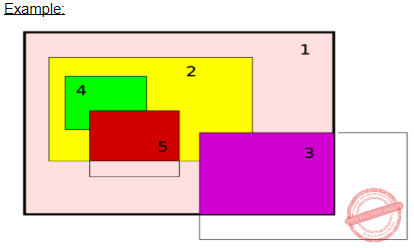Question 69
Which of the following statement(s) is/are True regarding ‘nice’ command of UNIX ?
I. It is used to set or change the priority of a process.
II. A process’s nice value can be set at the time of creation.
III. ‘nice’ takes a command line as an argument.
 A I, II only B II, III only C I, II, III D I, III only
Operating-Systems       UNIX-Operating-System
Question 69 Explanation:
Nice directly maps to a kernel call of the same name. nice is used to invoke a utility or shell script with a particular priority, thus giving the process more or less CPU time than other processes. A niceness of −20 is the highest priority and 19 is the lowest priority. The default niceness for processes is inherited from its parent process and is usually 0.
 Question 70
Let ν(x) mean x is a vegetarian, m(y) for y is meat, and e(x, y) for x eats y. Based on these, consider the following sentences :
I. ∀x ν(x ) ⇔ (∀y e(x, y) ⇒ ¬m(y))
II. ∀x ν(x ) ⇔ (¬(∃ym(y) ∧e(x, y)))
III. ∀x (∃y m(y) ∧e(x, y)) ⇔ ¬ν(x)
One can determine that
 A Only I and II are equivalent sentences B Only II and III are equivalent sentences. C Only I and III are equivalent sentence D I, II, and III are equivalent sentences.
Engineering-Mathematics       Propositional-Logic
 Question 71
Match each Artificial Intelligence term in List-I that best describes a given situation in List-IIA I-d, II-a, III-b, IV-c B I-d, II-c, III-a, IV-b C I-d, II-c, III-b, IV-a D I-c, II-d, III-a, IV-b
Artificial-intelligence       Knowledge-representation
Question 71 Explanation:
Semantic network→ A method of knowledge representation that uses a graph
Frame→ A data structure representing stereotypical knowledge
Declarative knowledge→ Knowledge about what to do as opposed to how to do it
Primitive→ A premise of a rule that is not concluded by any rule
 Question 72
In Artificial Intelligence , a semantic network
 A is a graph-based method of knowledge representation where nodes represent concepts and arcs represent relations between concepts. B is a graph-based method of knowledge representation where nodes represent relations between concepts and arcs represent concepts. C represents an entity as a set of slots and associated rules. D is a subset of rst-order logic.
Artificial-intelligence       Semantic-Network
Question 72 Explanation:
→ In Artificial Intelligence , a semantic network is a graph-based method of knowledge representation where nodes represent concepts and arcs represent relations between concepts.
→ Semantic networks are used in natural language processing applications such as semantic parsing and word-sense disambiguation.
 Question 73
Criticism free idea generation is a factor of _____.
 A Decision Support System B Group Decision Support System C Enterprise Resource Support System D Artificial Intelligence
Big-Data       Group-Decision-Support-System
Question 73 Explanation:
Criticism free idea generation is a factor of Group Decision Support System.
 Question 74
Consider the following logical inferences :
I1 : If it is Sunday then school will not open.
The school was open.
Inference : It was not Sunday.
I2 : If it is Sunday then school will not open.
It was not Sunday.
Inference : The school was open.
Which of the following is correct ?
 A Both I1 and I2 are correct inferences. B I1 is correct but I2 is not a correct inference. C I1 is not correct but I2 is a correct inference. D Both I1 and I2 are not correct inferences.
Engineering-Mathematics       Propositional-Logic
 Question 75
Which formal system provides the semantic foundation for Prolog?
 A Predicate calculus B Lambda calculus C Hoare logic D Propositional logic
Artificial-intelligence       Prolog
Question 75 Explanation:
Predicate calculus provides the semantic foundation for Prolog.
There are 75 questions to complete.